# 七夕，阿里云AI帮你算算你的撩妹战斗值

2020/08/25 13:07

"用姓名测试爱情，80%准确率！ “

“俗话说，名如其人，缘分就是人生的后半生。”

“心动不如行动，来一个属于自己的名字配对缘分测试吧！”

import pandas as pd
df = pd.read_csv('Speed Dating Data.csv', encoding='gbk')
print(df.shape)


percent_missing = df.isnull().sum() * 100 / len(df)
missing_value_df = pd.DataFrame({
'column_name': df.columns,
'percent_missing': percent_missing
})
missing_value_df.sort_values(by='percent_missing')


# 多少人通过Speed Dating找到了对象
plt.subplots(figsize=(3,3), dpi=110,)
# 构造数据
size_of_groups=df.match.value_counts().values

single_percentage = round(size_of_groups/sum(size_of_groups) * 100,2)
matched_percentage = round(size_of_groups/sum(size_of_groups)* 100,2)
names = [
'Single:' + str(single_percentage) + '%',
'Matched' + str(matched_percentage) + '%']

# 创建饼图
plt.pie(
size_of_groups,
labels=names,
labeldistance=1.2,
colors=Pastel1_3.hex_colors
)
plt.show()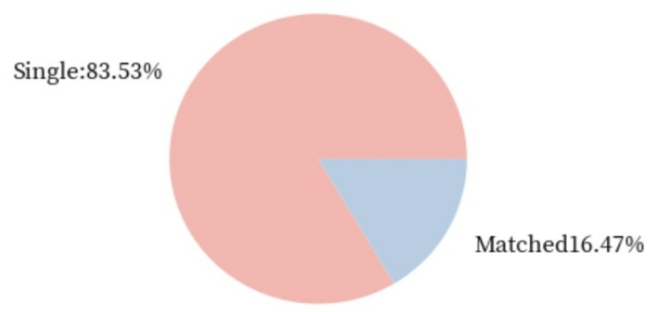df[df.gender == 0]


# 多少女生通过Speed Dating找到了对象
plt.subplots(figsize=(3,3), dpi=110,)
# 构造数据
size_of_groups=df[df.gender == 0].match.value_counts().values # 男生只需要吧0替换成1即可

single_percentage = round(size_of_groups/sum(size_of_groups) * 100,2)
matched_percentage = round(size_of_groups/sum(size_of_groups)* 100,2)
names = [
'Single:' + str(single_percentage) + '%',
'Matched' + str(matched_percentage) + '%']

# 创建饼图
plt.pie(
size_of_groups,
labels=names,
labeldistance=1.2,
colors=Pastel1_3.hex_colors
)
plt.show()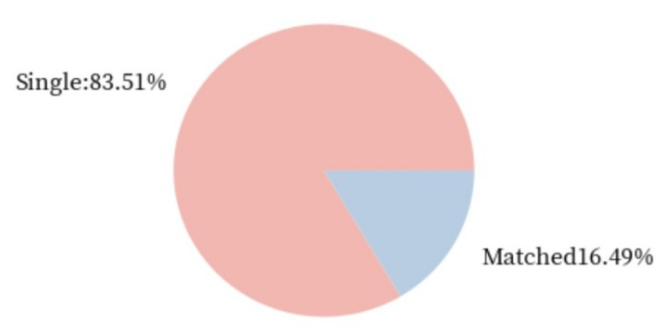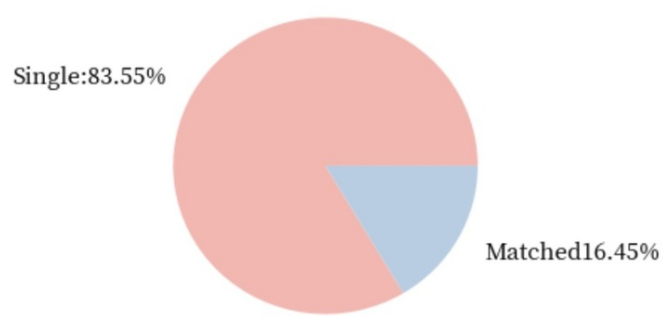# 年龄分布
age = df[np.isfinite(df['age'])]['age']
plt.hist(age,bins=35)
plt.xlabel('Age')
plt.ylabel('Frequency')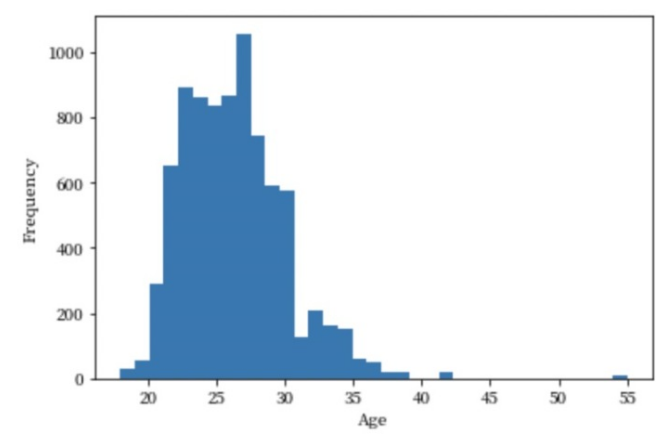date_df = df[[
'iid', 'gender', 'pid', 'match', 'int_corr', 'samerace', 'age_o',
'race_o', 'pf_o_att', 'pf_o_sin', 'pf_o_int', 'pf_o_fun', 'pf_o_amb',
'pf_o_sha', 'dec_o', 'attr_o', 'sinc_o', 'intel_o', 'fun_o', 'like_o',
'prob_o', 'met_o', 'age', 'race', 'imprace', 'imprelig', 'goal', 'date',
'go_out', 'career_c', 'sports', 'tvsports', 'exercise', 'dining',
'museums', 'art', 'hiking', 'gaming', 'clubbing', 'reading', 'tv',
'theater', 'movies', 'concerts', 'music', 'shopping', 'yoga', 'attr1_1',
'sinc1_1', 'intel1_1', 'fun1_1', 'amb1_1', 'attr3_1', 'sinc3_1',
'fun3_1', 'intel3_1', 'dec', 'attr', 'sinc', 'intel', 'fun', 'like',
'prob', 'met'
]]

# heatmap
plt.subplots(figsize=(20,15))
ax = plt.axes()
ax.set_title("Correlation Heatmap")
corr = date_df.corr()
sns.heatmap(corr,
xticklabels=corr.columns.values,
yticklabels=corr.columns.values)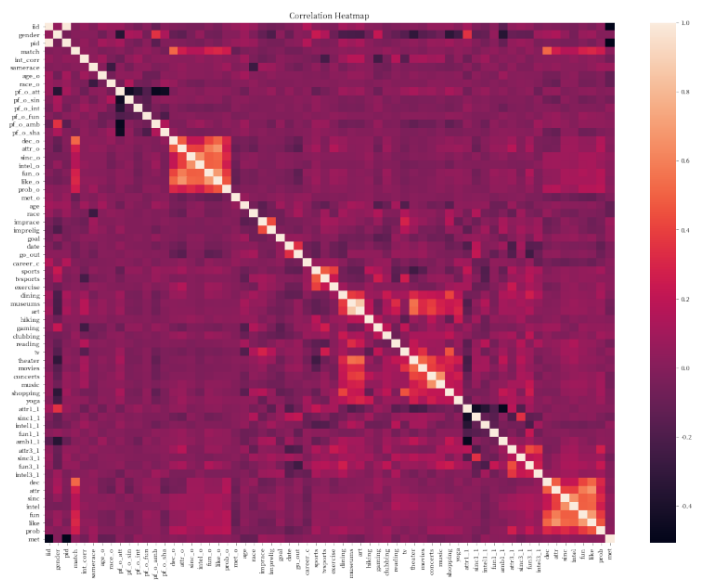# preparing the data
clean_df = df[['attr_o','sinc_o','intel_o','fun_o','amb_o','shar_o','match']]
clean_df.dropna(inplace=True)
X=clean_df[['attr_o','sinc_o','intel_o','fun_o','amb_o','shar_o',]]
y=clean_df['match']

oversample = imblearn.over_sampling.SVMSMOTE()
X, y = oversample.fit_resample(X, y)

# 做训练集和测试集分割
X_train, X_test, y_train, y_test = train_test_split(X, y, test_size=0.2, random_state=0, stratify=y)

# logistic regression classification model
model = LogisticRegression(C=1, random_state=0)
lrc = model.fit(X_train, y_train)
predict_train_lrc = lrc.predict(X_train)
predict_test_lrc = lrc.predict(X_test)
print('Training Accuracy:', metrics.accuracy_score(y_train, predict_train_lrc))
print('Validation Accuracy:', metrics.accuracy_score(y_test, predict_test_lrc))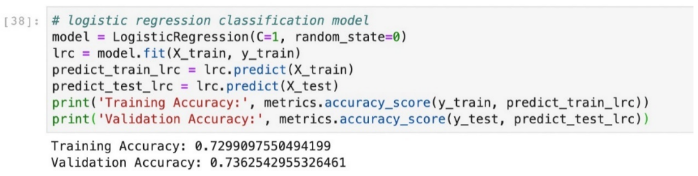0 评论
0 收藏
0